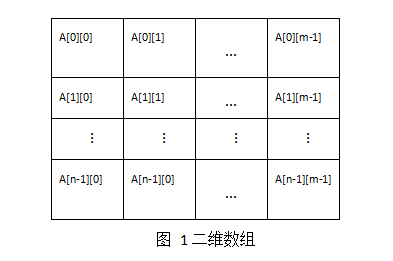• 之前跟同学讨论过numpy数组占用空间大小问题，但是今天给忘了，又重新试验了一下，主要是利用sys模块的getsizeof函数，使用的版本是 Python3.5。记录下来，以备后忘。 问题 一个空的numpy数组对象占用多大...
之前跟同学讨论过numpy数组的占用空间大小问题，但是今天给忘了，又重新试验了一下，主要是利用sys模块的getsizeof函数，使用的版本是 Python3.5。记录下来，以备后忘。

问题
一个空的numpy数组对象占用多大空间。一个int32、int64、float32、float64数占用多大空间。

代码
import numpy as np
import sys

# 32位整型
ai32 = np.array([], dtype=np.int32)
bi32 = np.arange(1, dtype=np.int32)
ci32 = np.arange(5, dtype=np.int32)

# 64位整型
ai64 = np.array([], dtype=np.int64)
bi64 = np.arange(1, dtype=np.int64)
ci64 = np.arange(5, dtype=np.int64)

# 32位浮点数
af32 = np.array([], dtype=np.float32)
bf32 = np.arange(1, dtype=np.float32)
cf32 = np.arange(5, dtype=np.float32)

# 64位浮点数
af64 = np.array([], dtype=np.float64)
bf64 = np.arange(1, dtype=np.float64)
cf64 = np.arange(5, dtype=np.float64)

print("size of 0 int32 number: %f" % sys.getsizeof(ai32))
print("size of 1 int32 number: %f" % sys.getsizeof(bi32))
print("size of 5 int32 numbers: %f" % sys.getsizeof(ci32), end='\n\n')

print("size of 0 int64 number: %f" % sys.getsizeof(ai64))
print("size of 1 int64 number: %f" % sys.getsizeof(bi64))
print("size of 5 int64 numbers: %f" % sys.getsizeof(ci64), end='\n\n')

print("size of 0 float32 number: %f" % sys.getsizeof(af32))
print("size of 1 float32 number: %f" % sys.getsizeof(bf32))
print("size of 5 float32 numbers: %f" % sys.getsizeof(cf32), end='\n\n')

print("size of 0 float64 number: %f" % sys.getsizeof(af64))
print("size of 1 float64 number: %f" % sys.getsizeof(bf64))
print("size of 5 float64 numbers: %f" % sys.getsizeof(cf64))

结果
size of 0 int32 number: 96.000000
size of 1 int32 number: 100.000000
size of 5 int32 numbers: 116.000000

size of 0 int64 number: 96.000000
size of 1 int64 number: 104.000000
size of 5 int64 numbers: 136.000000

size of 0 float32 number: 96.000000
size of 1 float32 number: 100.000000
size of 5 float32 numbers: 116.000000

size of 0 float64 number: 96.000000
size of 1 float64 number: 104.000000
size of 5 float64 numbers: 136.000000
以上结果说明：
一个空的 numpy 数组，无论什么类型，都是占用 96 个字节（byte）。一个 int32 和一个 float32 都是占用 4 个字节，而64位的都占用 8 个字节。
其他类型占用空间大小可以采用类似代码进行测试。
此外，注意 sys.getsizeof() 函数返回的是 这个对象所占用的空间大小，对于数组来说，除了数组中每个值占用空间外，数组对象还会存储数组长度、数组类型等其他信息。而如果只想要获取数组中存储的值的占用空间大小，可以使用 numpy.ndarray.nbytes ，使用 numpy.ndarray.itemsize 获取数组中每个值的占用空间大小。
ci32.nbytes    # 20
ci32.itemsize    # 4

END
展开全文python 内存 numpy
• 我们在前面的php高效写法提到，尽量不要复制变量，特别是...下面我们可以粗略的估算PHP数组占用内存的大小,首先我们测试1000个元素的整数占用的内存：  echo memory_get_usage() , ''; $start = memor  我们在前面的php高效写法提到，尽量不要复制变量，特别是数组。一般来说，PHP数组的内存利用率只有 1/10, 也就是说，一个在C语言里面100M 内存的数组，在PHP里面就要1G。下面我们可以粗略的估算PHP数组占用内存的大小,首先我们测试1000个元素的整数占用的内存： <?php echo memory_get_usage() , '<br>';$start = memory_get_usage();      $a = Array(); for ($i=0; $i<1000;$i++) {      $a[$i] = $i +$i;      }      $mid = memory_get_usage(); echo memory_get_usage() , '<br>'; for ($i=1000; $i<2000;$i++) {      $a[$i] = $i +$i;      }      $end = memory_get_usage(); echo memory_get_usage() , '<br>'; echo 'argv:', ($mid - $start)/1000 ,'bytes' , '<br>'; echo 'argv:',($end - $mid)/1000 ,'bytes' , '<br>'; 输出是: 353352 437848 522024 argv:84.416bytes argv:84.176bytes 大概了解1000 个元素的整数数组需要占用 82k 内存，平均每个元素占用 84 个字节。而纯 C 中整体只需要 4k(一个整型占用4byte * 1000 )。memory_get_usage() 返回的结果并不是全是被数组占用了，还要包括一些 PHP 运行本身分配的一些结构，可能用内置函数生成的数组更接近真实的空间: <?php$start = memory_get_usage();      $a = array_fill(0, 10000, 1);$mid = memory_get_usage(); //10k elements array;      echo 'argv:', ($mid -$start )/10000,'byte' , '<br>';      $b = array_fill(0, 10000, 1);$end = memory_get_usage(); //10k elements array;      echo 'argv:', ($end -$mid)/10000 ,'byte' , '<br>';

得到: argv:54.5792byte argv:54.5784byte
从这个结果来看似乎一个数组元素大约占用了54个字节左右。
首先看一下32位机C语言各种类型占用的字节：

#include "stdafx.h"  //#include <stdio.h>     int main() {          printf("int:%d\nlong:%d\ndouble:%d\nchar*:%d\nsize_t:%d\n",           sizeof(int), sizeof(long),           sizeof(double), sizeof(char *),           sizeof(size_t));      return   0;   }int:4 long:4 double:8 har*:4 size_t:4 在PHP中都使用long类型来代表数字，没有使用int类型 大家都明白PHP是一种弱类型的语言，它不会去区分变量的类型，没有int float char *之类的概念。 我们看看php在zend里面存储的变量，PHP中每个变量都有对应的 zval， Zval结构体定义在Zend/zend.h里面，其结构:

typedef struct _zval_struct zval;  struct _zval_struct {      /* Variable information */      zvalue_value value;     /* The value 1 12字节(32位机是12，64位机需要8+4+4=16) */      zend_uint refcount__gc; /* The number of references to this value (for GC) 4字节 */      zend_uchar type;        /* The active type 1字节*/      zend_uchar is_ref__gc;  /* Whether this value is a reference (&) 1字节*/  };

PHP使用一种UNION结构来存储变量的值,即zvalue_value 是一个union，UNION变量所占用的内存是由最大
成员数据空间决定。

typedef union _zvalue_value {      long lval;                  /* long value */      double dval;                /* double value */      struct {                    /* string value */          char *val;          int len;      } str;       HashTable *ht;              /* hash table value */      zend_object_value obj;      /*object value */  } zvalue_value;

最大成员数据空间是struct str，指针占*val用4字节，INT占用4字节，共8字节。

struct zval占用的空间为8+4+1+1 = 14字节，

其实呢，在zval中数组，字符串和对象还需要另外的存储结构，数组则是一个 HashTable:
HashTable结构体定义在Zend/zend_hash.h.

typedef struct _hashtable {      uint nTableSize;//4      uint nTableMask;//4      uint nNumOfElements;//4      ulong nNextFreeElement;//4      Bucket *pInternalPointer;   /* Used for element traversal 4*/      Bucket *pListHead;//4      Bucket *pListTail;//4      Bucket **arBuckets;//4      dtor_func_t pDestructor;//4      zend_bool persistent;//1      unsigned char nApplyCount;//1      zend_bool bApplyProtection;//1  #if ZEND_DEBUG      int inconsistent;//4  #endif  } HashTable;

HashTable 结构需要 39 个字节，每个数组元素存储在 Bucket 结构中:

typedef struct bucket {      ulong h;    /* Used for numeric indexing                4字节 */      uint nKeyLength;    /* The length of the key (for string keys)  4字节 */      void *pData;        /* 4字节*/      void *pDataPtr;         /* 4字节*/      struct bucket *pListNext;  /* PHP arrays are ordered. This gives the next element in that order4字节*/      struct bucket *pListLast;  /* and this gives the previous element           4字节 */      struct bucket *pNext;      /* The next element in this (doubly) linked list     4字节*/      struct bucket *pLast;      /* The previous element in this (doubly) linked list     4字节*/      char arKey;            /* Must be last element   1字节*/  } Bucket;

Bucket 结构需要 33 个字节，键长超过四个字节的部分附加在 Bucket 后面，而元素值很可能是一个 zval 结构，另外每个数组会分配一个由 arBuckets 指向的 Bucket 指针数组， 虽然不能说每增加一个元素就需要一个指针，但是实际情况可能更糟。这么算来一个数组元素就会占用 54 个字节，与上面的估算几乎一样。
一个空数组至少会占用 14(zval) + 39(HashTable) + 33(arBuckets) = 86 个字节，作为一个变量应该在符号表中有个位置，也是一个数组元素，因此一个空数组变量需要 118 个字节来描述和存储。从空间的角度来看，小型数组平均代价较大，当然一个脚本中不会充斥数量很大的小型数组，可以以较小的空间代价来获取编程上的快捷。但如果将数组当作容器来使用就是另一番景象了，实际应用经常会遇到多维数组，而且元素居多。比如10k个元素的一维数组大概消耗540k内存，而10k x 10 的二维数组理论上只需要 6M 左右的空间，但是按照 memory_get_usage 的结果则两倍于此，[10k,5,2]的三维数组居然消耗了23M，小型数组果然是划不来的。
展开全文php
• System.out.println("用一维数组存储占用内存总量为：" + memory1); long endTime1 = System.currentTimeMillis(); // 获取结束时间 System.out.println("程序运行时间： " + (endTime1 - startTime1) ...
• 数组中元素存储位置问题 设有 一个二维数组 A [m][n] ，假设 A ...数组在内存中的存储空间是连续的，也就是说如果一个一维数组的第一个元素存放位置在100D，那么第二个元素就存放在101D。 二维数组可以看做...
设有 一个二维数组 A [m][n] ，假设 A  存放位置在 644D ， A  存放位置在 676D，每个元素占一个空间，问 A 存放在什么位置？注D表示用 10 进制表示。
解析：
数组在内存中的存储空间是连续的，也就是说如果一个一维数组的第一个元素存放位置在100D，那么第二个元素就存放在101D。
二维数组可以看做是一个矩阵，在存储问题上可以有按行顺序存储和按列顺序存储。假设这个数组是一个n行m列的二维数组，如下图所示：如果按行优先顺序存储的话，每个元素占一个空间，第一个元素A的存储位置为644D则:
第一行元素的存储位置位: 644D , 645D … (644+(m-1))D；
第二行的存储位置为：(644+m)D，(644+m+1)D … (644+2m-1)D;
第三行的存储位置为：(644+2m)D，(644+2m+1)D … (644+3m-1)D;
依次类推
第n行存储位置为:(644+(n-1)m)D,(644+(n-1)m+1)D … (644+nm-1)D。
题中告知A的存储位置为676D，则(644+2m+2)D=676D,可以的解出m=15。所以可以有A的存储位置为：(644+3m+3)=692D，即A的存储位置为692D。
如果按列优先顺序存储的话，每个元素占一个空间，第一个元素A的存储位置为644D则:
第一列元素的存储位置位: 644D , 645D … (644+(n-1))D；
第二列的存储位置为：(644+n)D，(644+n+1)D … (644+2n-1)D;
第三列的存储位置为：(644+2n)D，(644+2n+1)D … (644+3n-1)D;
依次类推
第n行存储位置为:(644+(m-1)n)D，(644+(m-1)n+1)D … (644+mn-1)D。
题中告知A的存储位置为676D，则(644+2n+2)D=676D,可以的解出n=15。所以可以有A的存储位置为：(644+3n+3)=692D，即A的存储位置为692D。
总结：
如果有一个二维数组A[n][m]，每个数组元素占用k个字节，第一个数组元素的存储地址是Loc(a)
按行优先顺序存放的数组元素a[i][j]（0 ≤ i ≤ n-1，0 ≤ j ≤ m-1）的存储地址为Loc(a)+(im+j)k;
按列优先顺序存放的数组元素a[i][j]（0 ≤ i ≤ n-1，0 ≤ j ≤ m-1）的存储地址为Loc(a)+(jn+i)k。
展开全文Java 数据结构 内存
• 详情请参考：【Software Myzone】：[url]http://www.firedragonpzy.com.cn/index.php/archives/1525[/url]
详情请参考：【Software Myzone】：[url]http://www.firedragonpzy.com.cn/index.php/archives/1525[/url]
展开全文c++
• 问题：在java中，一维数组和二维数组在数据量一样的情况下，开辟的内存大小是怎样的？ 一、尝试阶段： 1、代码一： public class OneArrayMemory{ public static void main(String[] args){ int num1 = 1024*...
• 数组存储结构，非压缩存储方式和压缩存储方式...数据结构 算法
• PHP中的数组到底占用多大的空间？ 简要：这篇文章我并不是按照原文逐字逐句的都翻译过来，其中略去了一些与本文知识点无关的内容，加入了一些个人理解，不过版权还是归原作者所有。文章主要讨论的是 PHP5.x 中的...
• 现在打算入库，但占用空间。 如果压缩了，对过去一小时内12个800*1000的数据就无法进行求和了？ 现在初步思路是想把数据经过压缩后存到mogodb数据库中。 如果求和取出来，再压。 请问大家有否一些更加好的解决方案。压缩 数据 数据库 解决方案
• 点击进入_更多_Java千百问1、数组的内存空间是何时分配的java中的数组是用来存储同一种数据类型的数据结构，一旦初始化完成，即所占的空间就已固定下来，初始化的过程就是分配对应内存空间的过程。即使某个元素被...分配内存
• 数组的两个主要的顺序存储方式，数组具有随机访问特点，要求能够依据下标计算出任一数组元素的存储地址（概念上的地址）：计算机主存的每个地址和存储内的每个字节是一一对应的，而每个字节间的排列顺序是线式的。...数据结构
• 1) 计算机会给二维数组分配一块连续的存储空间 2) 数组名表示该二维数组的首地址，从首地址开始，依次存入第一行、第二行…. 3) 每一行的存储方式，从首地址开始，依次存入第一个元素、第二个元素….. 4) 每...c语言 内存管理
• ## 数组存储压缩原理

千次阅读 2019-06-03 20:28:38
文章目录1.存储单元2.存储方式3.数组存储压缩 1.存储单元 内存分为若干个存储单元，计算机记录着这些存储单元的首地址。一个存储单元的大小通常为4KB。 2.存储方式 计算机只保留每个存储单元的首...3.数组存储压缩...
• 语言中字符数组存储 程序片段 :   void mstrcat(char *s, char *t) { s += strlen(s); for(;*t!='\0';*s++=*t++); *s = '\0'; }     这是一个字符数组连接的函数 . (一)在测试程序中声明如下 : ...C C++ C# D语言 VC++
• ## Java 数组存储机制

千次阅读 2018-10-08 14:32:12
数组用来存储类型相同的一组数据，一旦初始化完成，其所占的空间也确定下来了，即使清除某个元素，其所占用空间仍然存在，即，数组的长度不能被改变，且数组只有在分配空间后才能使用。 数组的初始化方式有两种，a...base
• http://blog.csdn.net/hguisu/article/details/7376705我们在前面的php高效写法提到，尽量不要复制变量，特别是数组。...下面我们可以粗略的估算PHP数组占用内存的大小,首先我们测试1000个元素的整数占用的内存：<?phpphp zend struct byte
• 数组存储压缩的本质就是为了节省空间，因为一个int类型占用一个存储单元会产生大量浪费。但是压缩存储并不意味着数组的长度可以是无限大‘，也是会有大小限制的，例如 ` int[] ms = new int; for(int ...压缩
• #include #include void main() { char *str(); ...答题时我通过筛除选项...因此，“sizeof(数组名)”表达的就是：数组元素*单个元素占用存储空间大小！ 比如上面的代码中，int3=1000*1=1000, int4=20*4=80.语言 c include
• 什么样的二叉树适合用数组存储？ 待着问题，然后来学习今天的内容 树 我们首先来看，什么是树？再完备的定义，都没有图直观。所以请看下图的树，都有什么特征。 里面的每个实心圆点被我们称之为节点，用来连线...二叉树
• 我们知道16进制的每个字符需要用4位二进制位来表示，Java中byte用二进制表示占用8位，也就是说一个byte可以存放两位16进制数，进一步说32位的MD5值，就可以存到16个字节中，这样一下就节约了一半的存储空间（实际...
• 数组存储时的压缩原理以及原因 这篇博客，都是以byte类型的数组为例子 数组是一个物理空间连续的结构，对于数组，内存只记住开头的地址，记全部对内存损耗大 在我们电脑内存里面，一般的话，内存单元都是占 4KB...压缩原理
• 数组array所占用空间为（）。 6个字节 C语言中的字符串用指向该串首个字符的指针表示。不保存长度信息，用'\0'来标识字符串的终止。 因此相当于 char arrays[] = {'C', 'h', 'i', 'n', 'a', '\0'}; 本来应该...
• 1) 计算机会给数组分配一块连续的存储空间。 2) 数组名代表数组的首地址，从首地址位置，依次存入数组的元素。 3) 每个元素占用相同的字节数[字节数取决于数组类型] 4) 数组的每个元素之间的地址连续。内存 C
• 如何使用vb语言中的函数系统来调用类型的大小？怎么得到数组元素所使用的类型占用了多少内存空间，和文件存储的匹配？
• 一个bool类型占用1个字节。int 类型占用4个字节。定义的数组的大小等于数组大小size*每个元素的大小。 测试方法: 用sizeof的方法可以测试出结果。数组也可以用这个来测试。 例如sizeof(int)等； int a; sizeof(a)...
• int findPosition(int *p,int low,int high)//传入数组,最小下标,最大下标 { int mid = p[low];//基准值随机选取,就选数组第一个好了,p[low]可以改动了 while(low )//左右同时向中间移动 { //先看 mid 右边...